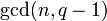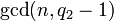# Number of conjugacy classes in projective general linear group of fixed degree over a finite field is PORC function of field size

## Statement

Suppose$n$ is a natural number. Then, there exists a PORC function$f$ of degree$n - 1$ such that, for any prime power$q$, the number of conjugacy classes in the projective general linear group$PGL(n,q)$ (i.e., the projective general linear group of degree$n$ over the finite field of size$q$) is$f(q)$.

A PORC function is a polynomial on residue classes -- it looks like different polynomial functions on different congruence classes modulo a particular number. In this case, we only need to consider congruence classes modulo$n$ to define the PORC function. In fact, for a field size of$q$, the polynomial depends only on the value$\operatorname{gcd}(n,q - 1)$.

## General observations

Below, we list some general observations about the PORC function in$q$ giving number of conjugacy classes in$PGL(n,q)$.

Item Value
Degree of polynomial$n - 1$ for all the polynomials
Leading coefficient of polynomial 1 for all the polynomials, i.e., each of the polynomials is monic
Factors of polynomial There are no common factors to all the polynomials
Coefficients of polynomial Is it also true that all coefficients of the polynomial are integers? (Seems so)
Are they always positive? (seems so)
Comparison between polynomials Suppose$q_1,q_2$ are prime powers such that$\operatorname{gcd}(n,q_1 - 1)$ divides$\operatorname{gcd}(n,q_2 - 1)$. Then, the polynomial that works on the residue class of$q_2$ is bigger than the polynomial that works on the residue class of$q_1$, where bigger means that the difference has positive leading coefficient.
In particular, the smallest polynomial corresponds to congruence classes that are 1 more than classes relatively prime to$n$, and the largest polynomial corresponds to the congruence class 1 mod$n$.

## Particular cases$n$ (degree of projective general linear group, also modulus to which we need to consider congruence classes)$n - 1$ (degree of PORC function) Possibilities for$\operatorname{gcd}(n,q - 1)$ Corresponding congruence classes mod$n$ for$q$ Corresponding polynomials in PORC function of$q$ giving number of conjugacy classes in$PGL(n,q)$ More information
1 0 1 1 1 the group is a trivial group
2 1 1
2
0
1$q + 1$$q + 2$
See element structure of projective general linear group of degree two over a finite field
3 2 1
3
0 or 2
1$q^2 + q$$q^2 + q + 2$
See element structure of projective general linear group of degree three over a finite field
4 3 1
2
4
0 or 2
3
1$q^3 + q^2 + q$$q^3 + q^2 + 2q + 1$$q^3 + q^2 + 2q + 3$
element structure of projective general linear group of degree four over a finite field